Courses

# Close Packing in Solids Class 12 Notes | EduRev

## JEE : Close Packing in Solids Class 12 Notes | EduRev

The document Close Packing in Solids Class 12 Notes | EduRev is a part of the JEE Course Chemistry for JEE.
All you need of JEE at this link: JEE

Close Packed Structures
In order to analyze close packing of constituent particles (ions, molecules or atoms) in a lattice, we get into the assumption that constituent particles (ions, molecules or atoms) are hard spheres of identical shape.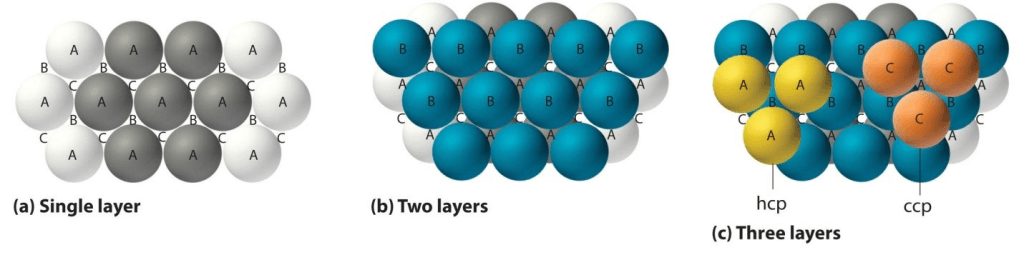Image 1: Different Types of Layers form closed packed structures

What is Close Packing?
The packing of constituent particles inside lattice in such a way that they occupy maximum available space in the lattice is known as Close Packing.
Close Packing is done in three ways, namely:
(a) One Dimensional Close Packing
(b) Two Dimensional Close Packing
(c) Three Dimensional Close Packing

(a) One Dimensional Close Packing
In one dimension close packing, the spheres are arranged in a row touching each other. In one-dimensional close packing, each sphere is in direct contact with two of its neighbor spheres. The number of nearest spheres to a particle in a lattice is called Coordination Number. Since there are two spheres in direct contact with the one sphere, the coordination number of one-dimensional close packing is 2.

(b) Two Dimensional Close Packing
Two-dimensional close packing is done by stacking rows of hard spheres one above the other. This can be done in two ways:
•  AAA type
•  ABA type

AAA Type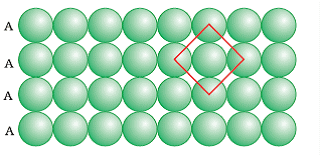Image 2: AA type packing

The packing in which one sphere touches two spheres placed in two different rows one above and one below is called AAA type close packing. The coordination number of AAA type two-dimensional close packing is 4. The AAA type is formed by placing one-dimensional row directly one above the other in both horizontal and vertical directions. It is also called two-dimensional square close packing as the rows of spheres when arranged in vertical and horizontal alignments form a square.

AB Type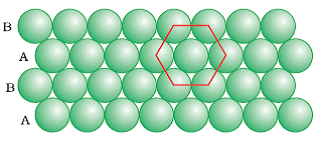Image 3: AB Type Packing

The packing in which the spheres in the second row are located in the depressions of the first row. The ABA type close packing is formed by placing one-dimensional row let’s say B type over the A type close packing and this series continues to form a two dimensional hexagonal. The coordination number of ABA Type packing is 6 as each sphere is in direct contact with 6 other spheres.
In ABA type close-packing we find triangular empty spaces called voids. These are of two types:
•  Apex of triangle pointing upwards
•  Apex of triangle pointing downwards

(c) Three Dimensional Close Packing

The formation of real lattices and structures take place through three-dimensional close packing. They are formed by stacking two-dimensional layers of spheres one above the other. This can be done by two ways:

• Three-dimensional close packing from two dimensional square close packed layers
• Three-dimensional close packing from two dimensional hexagonal close packed layers

Three-dimensional close packing from two-dimensional close packed layers
Formation of three-dimensional close packing can be done by placing the second square closed packing exactly above the first one. In this close packing, the spheres are aligned properly in horizontally and vertically. Similarly, by placing more layers one above the other, we can obtain a simple cubic lattice. The unit cell of the simple cubic lattice is called the primitive cubic unit cell.
Three-dimensional close packing from two dimensional hexagonal close packed layers
Three-dimensional close packing can be formed with the help of two-dimensional hexagonal packed layers in two ways:
•  Stacking the second layer over the first layer
•  Stacking the third layer over the second layer

Stacking the second layer over the first layer
Suppose we take two hexagonal close packed layer ‘A’ and place it over the second layer B ( as both layers have different alignment of spheres) such that spheres of the second layer are placed in the depressions of the first layer. We observe that a tetrahedral void is formed when a sphere of the second layer is right above the void (empty space) of the first layer. Adding further we notice octahedral voids at the points where the triangular voids of the second layer are placed right triangular voids of the first one in such a way that triangular space doesn't overlap. Octahedral voids are bordered by six spheres.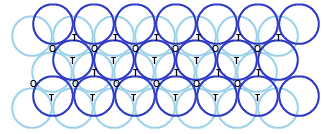Image 4: Types of voids in three-dimensional closed packing

If there are ‘N’ closed spheres, then:
•  Number of Octahedral Voids equals to “N”
•  Number of Tetrahedral Voids equals to “ 2N”
Stacking the third layer over the second layer
There are two possible ways of placing the third layer over the second layer:

(1) By Covering Tetrahedral Voids
(2) By Covering Octahedral Voids

(1) Covering Tetrahedral Voids
In this kind of three-dimensional packing, the spheres of the third layer are aligned right above the spheres of the first layer. If we name the first layer as A and second layer as B, then the pattern will be ABAB… so far and so forth. The structure formed is also called hexagonal close-packed structure also known as HCP.

(2) Covering Octahedral Voids
In this kind of packing the third layer, spheres are not placed with either of the second layer or first layer. If we name the first layer as A, second as B and then the third layer will be C (as it is now a different layer) then the pattern will be ABCABC… The structure formed is also called cubic closed packed (ccp) or face-centred packed cubic structure (fcc). For Example metals like copper and iron crystallize in the structure.
The coordination number in both cases will be 12 as each sphere in the structure is in direct contact with 12 other spheres. The packing is highly efficient and around 74% of the crystal is completely occupied.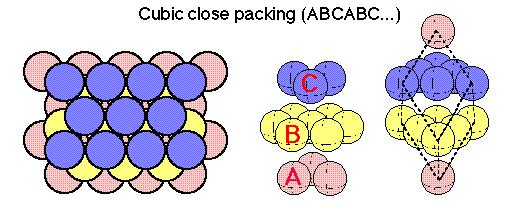Image 5: ABC Type of Close Packing

Offer running on EduRev: Apply code STAYHOME200 to get INR 200 off on our premium plan EduRev Infinity!

## Chemistry for JEE

223 videos|452 docs|334 tests

,

,

,

,

,

,

,

,

,

,

,

,

,

,

,

,

,

,

,

,

,

;